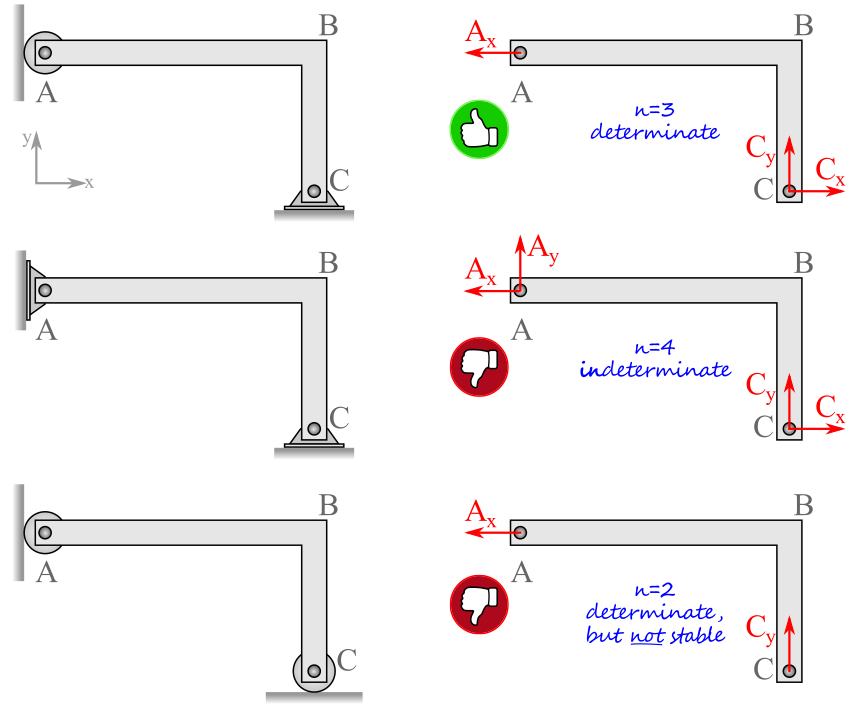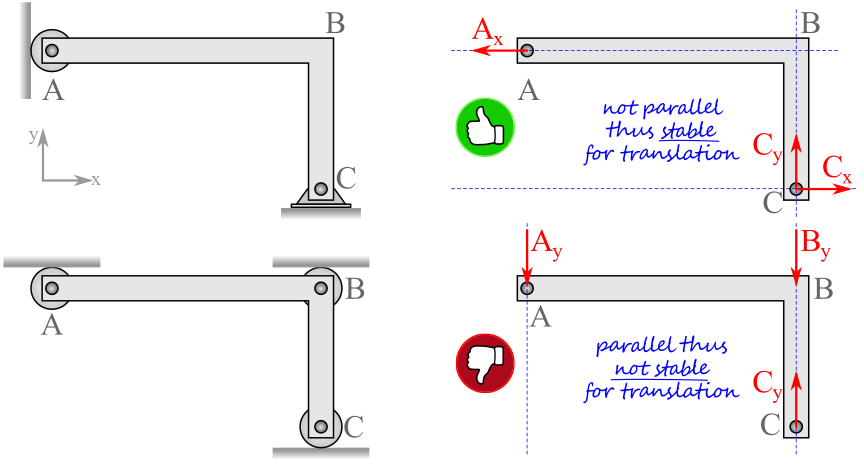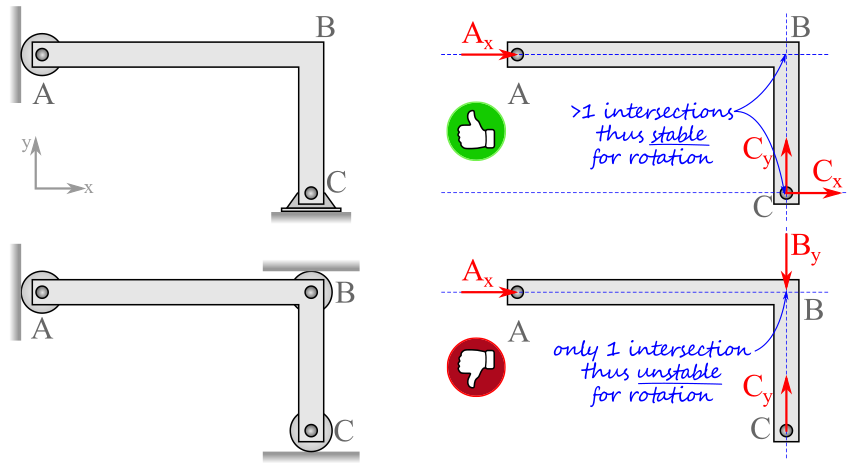# Engineering Statics: Open and Interactive

## Section5.6Stability and Determinacy

### Determinate vs. Indeterminate.

A static system is determinate if it is possible to find the unknown reactions using the methods of statics, that is, by using equilibrium equations, otherwise it is indeterminate.
In order for a system to be determinate the number of unknown force and moment reaction components must be less than or equal to the number of independent equations of equilibrium available. Each equilibrium equation derives from a degree of freedom of the system, so there may be no more unknowns than degrees of freedom. This means that we can determine no more than three unknown reaction components in two-dimensional systems and no more than six in three-dimensional systems.
An indeterminate system with fewer reaction components than degrees of freedom is under-constrained and therefore unstable. On the other hand, if there are more reaction components than degrees of freedom, the system is both over-constrained and indeterminate. In terms of force and moment equations, there are more unknowns than equilibrium equations so they can’t all be determined. This is not to say that it is impossible to find all reaction force on an over-constrained system, just that you will not learn how to find them in this course.

### Stable vs. Unstable.

A body in equilibrium is held in position by its supports, which restrict the body’s motion and counteract the applied loads. When there are sufficient supports to restrain a body from moving, we say that the body is stable. A stable body is prevented from translating and rotating in all directions. A body which can move is unstable even if it is not currently moving, because the slightest change in load may take it out of equilibrium and initiate motion. Stability is loading independent i.e. a stable body is stable for any loading condition.

### Rules to Validate a Stable and Determinate System.

There are three rules to determine if a system is both stable and determinate. While, the rules below can technically be checked in any order, they have been sorted from the quickest to the most time consuming to speed up your analysis.
Rule 1: Are there exactly three reaction components on a two-dimensional body?
If YES, the system is determinate.
If NO, the system is indeterminate or not stable.Rule 2: Are all the reaction force components parallel to one another?
If YES, the system is unstable for translation.
If NO, the system is stable for translation.Rule 3: Do the lines of action of the reaction forces intersect at a single point?
If YES, the system is unstable for rotation about the single intersection point.
If NO, the system is stable for rotation.